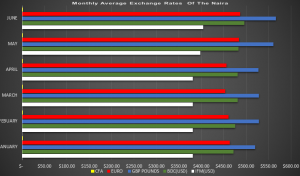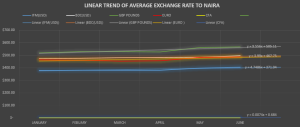AVERAGE MONTHLY EXCHANGE TO NAIRA ANALYSIS

The monthly average exchange rate of the naira per unit of foreign currency shows how naira fairs in relation to selected currency.  The analysis below visually described  the correlation amongst these variables as well as there trend lines

a simple chart was used to describe the size of this averages over the the first 6 months of the year 2021 , then a linear graph explained the trend and equation line of the exchange rates.

Fig 1.0Fig 1.0 describes how naira faired in respect to CFA, DOLLARS DAS/WDAS = Dutch Auction System/Wholesale Dutch Auction System , DOLLARS IFEM is Inter-bank Foreign Exchange Market, BDC is Bureau de Change, EURO,POUNDS, in the Foreign Exchange Market.

CFA notably has been exchanged at Naira at a close rate with IFEM DOLLARS close .

Fig 1.1Fig 1.1 shows the linear relationship between the variables as well as each equation and there intercept over time period of 6 months , the graph visualizes the trend of the average naira exchange rate.

NOTE: In April 2021 there was a drop in average exchange rate for GBD pounds to Naira and there was also a drop in EURO in April thus Naira did considerably well in April  foreign exchange market, However CFA remains slightly stable over the past 6 months on 2021 with the equation y = 0.0074x + 0.684 a change in naira will necessitate a slight change in CFA compared to other equations;

y = 4.7486x + 371.04

y = 5.3363x + 448.91

y = 3.99x + 467.75

y = 9.556x + 505.11

USING R  PROGRAMMING LANGUAGE

RESULTS FOR CORRELATION

Pearson’s product-moment correlation

data: AVG\$`BDC(USD)` and AVG\$POUNDS
t = 2.9669, df = 4, p-value = 0.04127
alternative hypothesis: true correlation is not equal to 0
95 percent confidence interval:
0.05390719 0.98076033
sample estimates:
cor
0.8291922

Pearson’s product-moment correlation

data: AVG\$EURO and AVG\$CFA
t = 4.7909, df = 4, p-value = 0.008706
alternative hypothesis: true correlation is not equal to 0
95 percent confidence interval:
0.4431189 0.9916840
sample estimates:
cor
0.9228181

Pearson’s product-moment correlation

data: AVG\$CFA and AVG\$POUNDS
t = 2.9501, df = 4, p-value = 0.04196
alternative hypothesis: true correlation is not equal to 0
95 percent confidence interval:
0.04923125 0.98058084
sample estimates:
cor
0.8277216

These results shows there is a strong correlation between all variables involved and they have a strong relationship with NAIRA

Thus this analysis can be used as a  basis to predict the trend to naira value in relation to selected variables